Back to Open Problems Group feed

===Open Problem #5===

Welcome to Open Problem #5! This is a problem I've picked, but I'd like Open Problem #6 to come from our suggestion thread which has been quiet lately. Please make some suggestions if you can!

If you're new here, you should start by reading some general information about this group.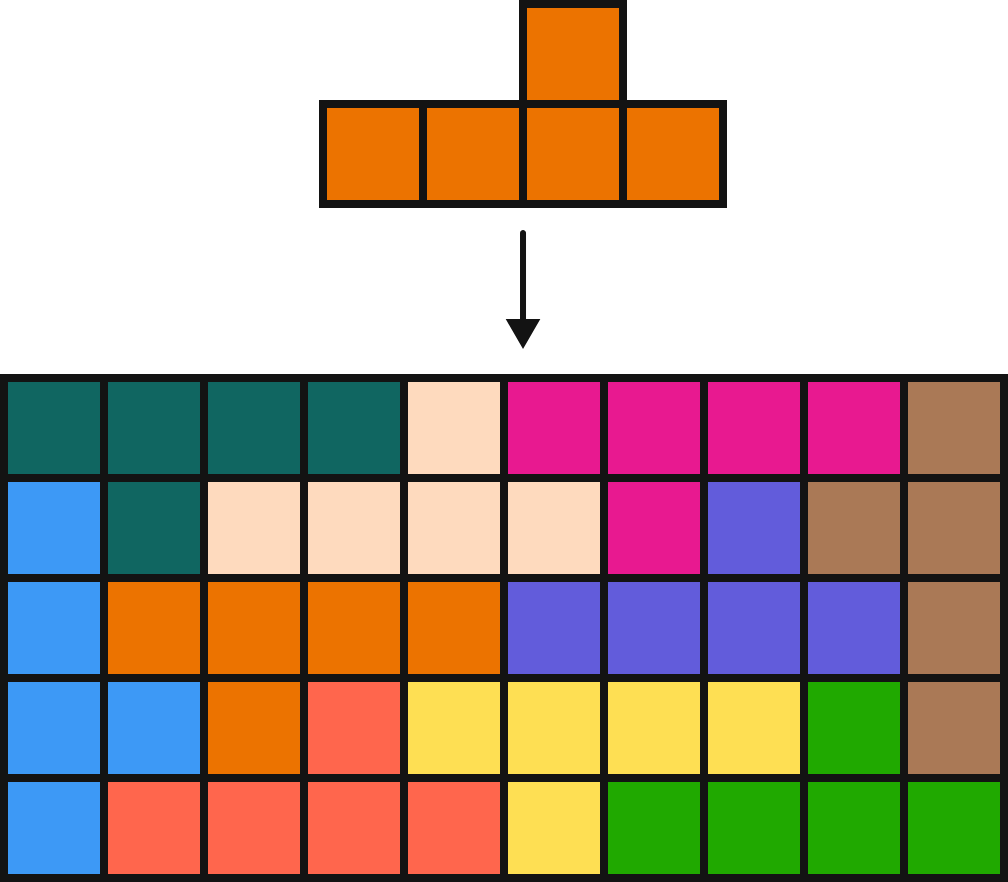Using copies of the polynomio above, you can use repeated copies to form a rectangle (with rotations and reflections allowed).

Using just copies of the polyomino below, is it possible to form them into a rectangle?The answer incidentally is thought to be "no", but nobody has proven it yet.

This problem of the week from a month ago gives something a flavor of the type of proof needed.

Typically, you figure out some value ("pointiness = 0" or the like) that all rectangles share. Then you find that adding a polynomio increases or decreases that value by some set amount, such that the target value is never reached.

This problem hasn't gotten a lot of attention, so it's a possible things are that simple. You may decide a different strategy altogether, though. Good luck!

Quick updates: Open Problem #2 has the paper done, I'm still looking into the possibility of publishing in a journal.

I will be soon contacting the Online Encyclopedia of Integer Sequences about Open Problem #4, which while not finding any "new" sequences found out information about existing ones and made connections between previously unrelated ones.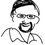Note by Jason Dyer
1 year, 6 months ago

This discussion board is a place to discuss our Daily Challenges and the math and science related to those challenges. Explanations are more than just a solution — they should explain the steps and thinking strategies that you used to obtain the solution. Comments should further the discussion of math and science.

When posting on Brilliant:

• Use the emojis to react to an explanation, whether you're congratulating a job well done , or just really confused .
• Ask specific questions about the challenge or the steps in somebody's explanation. Well-posed questions can add a lot to the discussion, but posting "I don't understand!" doesn't help anyone.
• Try to contribute something new to the discussion, whether it is an extension, generalization or other idea related to the challenge.
• Stay on topic — we're all here to learn more about math and science, not to hear about your favorite get-rich-quick scheme or current world events.

MarkdownAppears as
*italics* or _italics_ italics
**bold** or __bold__ bold

- bulleted
- list

• bulleted
• list

1. numbered
2. list

1. numbered
2. list
Note: you must add a full line of space before and after lists for them to show up correctly
paragraph 1

paragraph 2

paragraph 1

paragraph 2

> This is a quote
This is a quote
# I indented these lines
# 4 spaces, and now they show
# up as a code block.

print "hello world"
# I indented these lines
# 4 spaces, and now they show
# up as a code block.

print "hello world"
MathAppears as
Remember to wrap math in $$...$$ or $...$ to ensure proper formatting.
2 \times 3 $2 \times 3$
2^{34} $2^{34}$
a_{i-1} $a_{i-1}$
\frac{2}{3} $\frac{2}{3}$
\sqrt{2} $\sqrt{2}$
\sum_{i=1}^3 $\sum_{i=1}^3$
\sin \theta $\sin \theta$
\boxed{123} $\boxed{123}$

Sort by:

I looked at the possible edges of the rectangle, starting wit labeling the eight possible orientations:Polyomino labels A - H

And then considered the sequence of orientations that could fill the edge:Empty edge L - R

Just using L and R to mark the start and end. The list of what orientations can follow each other in sequence without holes is: L -> ABFH A -> ABCDEFGHR B -> ABCEFGHR C -> B D -> AB E -> ABFHR F -> AB G -> ABFHR H -> AB A lot of these combinations leave squares that cannot be filled with other polyominos, though:Blocked Squares

Since AG and BG do not fit, by symmetry, HB and HA don't work, so G and H are eliminated. This also means that LB and AR don't fit, since the first and last polyomino in one edge are part of the next edge. Also by symmetry BB and EB are not possible. This leaves: L->AF / A->CDE / B->ACEFR / C->B / D->AB / E->AFR / F->AB Which can be summarized in a graph:Edge graph

This graph generates a language that codes the flat edges that can be filled with the polyomino. It can be seen that this language is closed under concatenation, so edges of any multiple can be made.

I only looked at sub sequences of length two to see if there were any squares that couldn't be filled deeper in the rectangle. The next step could be to use this to expand to length three sub strings, eliminating any that don't work, thereby getting a more precise language for the edge. Then length four, five, etc. This might lead to a contradiction, or to an edge that works. I will start working on the length three sub strings.

edit: sorry, those pictures are a lot bigger than I expected

- 1 year, 5 months ago

I like bigger. Easy to read.

- 1 year, 5 months ago

Before we tackle this particular problem, I think it would be advantageous to take a look into what type of polynomio make rectangles and which don't. I'm guessing some sort of pattern will emerge that will help us on our way.

- 1 year, 6 months ago

Sounds good! Do you want to try some?

Staff - 1 year, 6 months ago

I wanna try!!!!!

- 1 year, 6 months ago

Here's a suggestion.

Go to the main Open Problems Group feed.

Click "Post Problem" and write up a problem you know the answer to (doing it this way will make it easier to post pictures, too). You could ask a single polynomio or a set and ask "how many of these can we make into rectangles?"

If we can get enough like this, we can start gathering on a wiki.

Staff - 1 year, 6 months ago

Thanks!!!!

- 1 year, 6 months ago

Maybe cutting up the polynomial into smaller polyominoes then seeing which are impossible would be useful.

- 1 year, 6 months ago

My idea was to try to construct, and continue until one of three things happens:

1. The rectangle is constructed, which would proof it's possible
2. A contradiction is reached, which would proof it's not possible
3. An infinite loop is reached, which would proof it's not possible

The furthest I was able to get was this. Assuming I didn't make any mistakes, it looks like the first two columns form an infinite loop, but I don't have a proof.

- 1 year, 6 months ago

Do you have a proof that yours is the only valid configuration for the corner?

- 1 year, 6 months ago

I suppose I could write a proof, but it would be a pretty long and messy proof, because it would include lots of cases and cases within cases. I started writing a bit, and just the first three polyomino already took 7 pages.

What I have so far: https://docs.google.com/document/d/10j13AQu4oOi6zBNfaX90hxJ_8nawAw7lGpEP9VhtOIc/edit?usp=sharing

- 1 year, 6 months ago

Could you please tell me how you are working with the polyminos in your computer or whatever. I'd like to do that and post something.

- 1 year, 6 months ago

Make square cells in Excel. Color them in to make the shapes.

- 1 year, 6 months ago

Thanks!

- 1 year, 6 months ago

Assuming it can form a rectangle of dimensions axb, then axb must be divisible by 10 and both a and b can be expressed as the sum of any combination of 7, 3, and 1. Just an observation that may help :)

- 1 year, 6 months ago

We could prove that this scenario is impossible.

- 1 year, 6 months ago

If you have a 3 at the edge, it has to be followed by a 7. These can be grouped together into a group of 10. Since adding 10 does not change the remainder by 2 or by 5, the remaining modular analysis would boil down to 7s and 1s; that on all four sides of the hypothetical rectangle.

- 1 year, 6 months ago

To fill in the corner of a rectangle with the given polynomio, the flat side of each polynomio must go up against the side of the rectangle (or there will be a gap). There are six ways to do this, as shown below.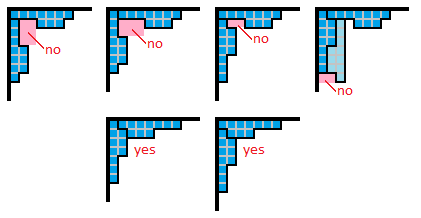When a 3-square-end meets another 3-square-end in the corner, then a 2 x 3 rectangular gap is formed that cannot be filled by any orientation of the polynomio (see the first two diagrams in the above picture). When a 3-square-end meets a 1-square-end in the corner, with the 3-square-end directly in the corner, then a 1 x 2 rectangular gap is formed that cannot be filled by any orientation of the polynomio (see the third diagram in the above picture). When a 3-square-end meets a 1-square-end in the corner, with the 1-square-end directly in the corner (see the fourth diagram in the above picture), then a 1 x 2 rectangular gap is formed near the corner that can only be filled by a certain orientation of a third polynomio (light blue), and this forms a 1 x 2 rectangular gap that cannot be filled by any combination of polynomios. This leaves only 2 possible ways to fill a corner - both when a 1-square-end meets another 1-square-end.

Therefore, if a rectangle does exist, the 1-square-end of one polynomio must meet another 1-square-end of another polynomio in all 4 of its corners.

(I was hoping to limit this further to either find a possible rectangle or prove that one does not exist, but unfortunately I was unable to get any further than this. Hopefully someone can use my work towards a complete solution.)

- 1 year, 6 months ago

So the edge must be some 10x+(9+a). I put in the a since we need it to be some (1+10b) so the edge is divisible by 10. So if the the 7 edge- 3 edge sequence cannot have a 1 edge in between (which would be a vertically flipped polymino), the rectangle cannot be constructed.

- 1 year, 6 months ago

The area must be divisible by 10, but not necessarily the edge - one edge could be divisible by 5 and the other could be divisible by 2.

- 1 year, 6 months ago

Agreed. But proving that one cannot put a 1-edge between a 7-edge and 3-edge will also disprove for divisibility by 2 since the edge would be 2(10x)+9, which is not divisible by 2.

- 1 year, 6 months ago

The 9 is from the extra 7-edge we always need to complete the 7-edge-3-edge sequence plus the two 1-edge at the corners.

- 1 year, 6 months ago

Sorry. The 9 is an 8. So it would no disprove by 2.

- 1 year, 6 months ago

Here are a lot of the "edge cases":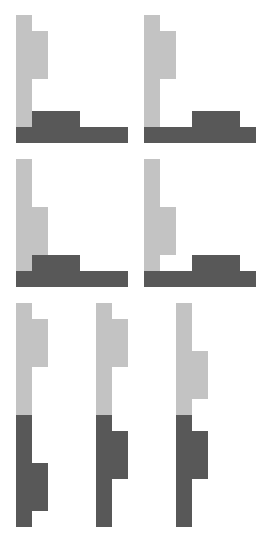By "edge case", I mean that these are the patterns that can be used on the edge of the rectangle, or in this case, the left edge (I've excluded the patterns that create completely enclosed holes). Of those edge cases, I'm pretty sure only 6 work, but I'm not too sure. Obviously, the second cases in the first and second row don't work, as they create holes that can't be filled by the shape. As far as I can tell, the only case in the third row that works is the third case, but I'm not too sure. I'm pretty sure that all 3 of the cases in the last row work.

- 1 year, 6 months ago

From my experimenting I would agree with all of your assessments.

- 1 year, 6 months ago

Also, as a thought, if we can split the polyomino into two or more separate polyominoes, then if we can't construct a rectangle using those two (possibly because of infinite loops), then it definitely isn't possible with the original polyomino.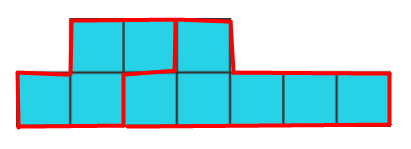Perhaps these two?

EDIT: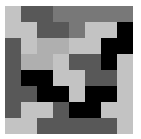Nevermind. I mean, this DOES make for a cool pattern, I guess...

- 1 year, 6 months ago

That's certainly worth a try, especially since the s-shape alone can't fill a rectangle.

- 1 year, 6 months ago

I tries messing around with the s one myself last night and came to that conclusion.

- 1 year, 6 months ago

Actually, I just had a thought - what if we try making a rectangle with the two pieces I cut the polyomino into, but this time, there has to be the same number of both pieces? This might actually be a hader problem, but if it turns out to be easy, and it ends up being impossible, then that's a possible solution.

- 1 year, 6 months ago

I have been doing some brute force searching by computer program. I not ready to give precise results but you may be interested in a flavour of what I am getting. The computer program is in java and at each stage tries to fit a piece on the next unoccupied square chosen as the left most column with unoccupied squars and then the square the bottom edge in that column. The pieces are tried in order and the prog first checks that the piece does not overlap an edge or previously place piece. If this check is satisfied, the prog then checks that the boundary does not leave one of a number of known "bays" that are known to be impossible to fill. If this check is satisfied the piece is placed on the board and we go onto the next iteration. If either check fails we try another piece or backtrack as appropriate. Obviously such an approach will not prove impossibility, and because of the exponential nature of the problem cannot work on large rectangles. Let the rectangle be of size X x Y where X is number of squares left to right, and Y up and down. Let X be large and as in my prog, tile the rectangle from the left, filling all the squares on left as we go. Then it is noticeable that even for reasonable large Y say up to around 100 then the number of columns that can be fully tiled from the left is never more than about six or so.
Consistent with this, the same way that others have noticed that bay on the bottom of say 1 deep by 4 wide is not tileable, there are many other bays that are not tileable, for example bays which are 4 or 5 squares deep are not tileable for a couple of dozen different widths. [I mean that a bay is tileable if it is possible to cover all its squares even if the tiles that do so reach over its boundary into the main area of the rectangle] In general the deeper the bay the wide it can be and still not tileable. A proper census of these impossible bays/boundary would speed up the search.

- 1 year, 5 months ago

Since trying to find the answer to this problem directly seems quite hard, I got the idea to try and answer the more general question first: which polyomino that are similar to the one in the problem can tile a rectangle?

First it's required to define 'similar' and find a systematic way to describe all those similar polyomino. I settled with this: Take the following polyomino: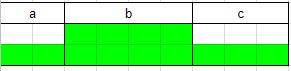• a describes how many columns with a single row of blocks there are on the left.
• b describes how many columns with two rows of blocks there are in the center.
• c describes how many columns with a single row of blocks there are on the right.

So the polyomino in the image has a = 2, b = 4 and c = 3.

For a and c the rule is that when the variable is positive, the blocks are in the bottom row. When the variable is negative, they are in the top row. b is only allowed to be positive.

Here are two more examples: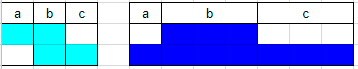The one on the left has a = -1, b = 1 and c = 1. The one on the right, which is also the main one of this open problem, has a = 1, b = 3 and c = 3.

Now let's define a function f(a, b, c) that takes in three variables that describe a polyomino as defined above. It returns 0 if it's impossible to construct a rectangle with the polyomino, 2 when it is possible, and 1 when it doesn't know whether or not the polyomino can tile a rectangle.

Let's look at how the function f behaves for different inputs.

f(a, b, c) = f(c, b, a) = f(-a, b, -c) = f(-c, b, -a)

Flipping around the a and c is the same as reflecting the polyomino horizontally. Multiplying a and c by -1 is the same as reflecting the polyomino vertically. Since all of those polyominoes are the same, the outputs of the function must be the same as well.

a = 0 or c = 0

In these cases it's very easy to tile a rectangle. Below it's shown why f(0, 2, 3) = 2.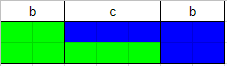Just rotate a polyomino 180 degrees and put them together. It doesn't matter what b is. a or c (the one that isn't zero) may be anything you want.

a and c have different signs

In this case it's impossible to tile a rectangle. To illustrate, let's look at why f(-1, 2, 1) = 0. This was mostly copied from the solution from this question.

Let's try to form a rectangle starting from the top-left corner. There are two ways to do it (the X represents the top-left of the rectangle):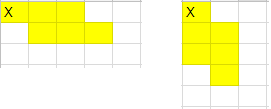Let's first look at the first way. There's a hole in the top-right, which needs to be filled up. There is only one way to do it.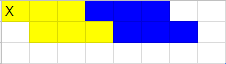But now there's a new hole in the top-right and there is again only one way to fill it. After that there's again a hole in the top-right, and it will keep going on like that forever.

The second way won't work for the same reason, except then the hole will always be in the bottom-left.

No matter what a, b and c are, as long as a and c have different signs, the argument for why it's not possible to tile a rectangle is mostly the same.

(a and c are both >= 2) or (a and c are both <= -2)

In this case it's also impossible to construct a rectangle. To illustrate, let's start with the concrete example f(2, 1, 3) and proof why it's not possible to construct a rectangle. To do this, I'm going for a proof by contradiction.

Assume it's possible to construct a rectangle with the (2, 1, 3) polyomino. Then this rectangle will have a top-left corner. There are four ways to fill this corner: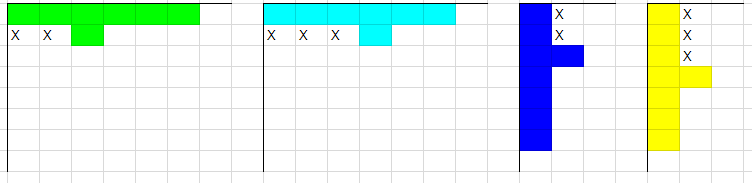Now look at the squares with an X. In all four scenarios it's impossible to fill all of the squares with an X. Therefore it's impossible to complete the rectangle.

No matter what a, b and c are, as long as a and c are both >= 2, it's always possible to point at multiple squares in the second row or second column that can't all get filled.

Since it has been mentioned earlier that f(a, b, c) = f(-a, b, -c) the same argument can get used to proof it's not possible to form a rectangle when a and c are both <= -2

Summarize the findings

Since f has three inputs, it's possible to imagine an infinitely large cube with all possible values for a, b and c. Here's a small section of it that shows what happens when b = 1: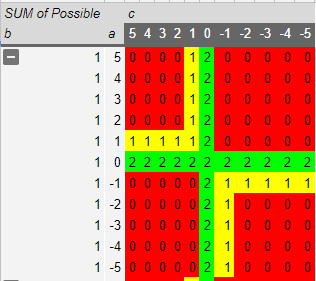Using just the details mentioned above, quite a lot of inputs of a, b and c can already get defined properly. But there are still a few holes. Those holes are of four types:

• a = 1 and c >= 1
• c = 1 and a >= 1
• a = -1 and c <= -1
• c = -1 and a <= -1

But since f(a, b, c) = f(c, b, a) = f(-a, b, -c) = f(-c, b, -a) all of these four types represent the same polyominoes. So it's possible to visualize all of the remaining polyominoes by looking just at the cases where a = 1, b >= 1 and c >= 1: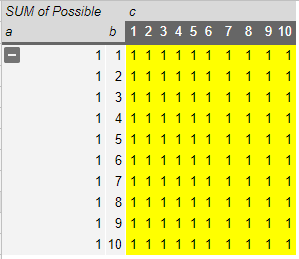I'm pretty sure I've also come up with a way to proof f(1, 1, 1) = 2, f(1, 2, 1) = 2 and f(1, >=3, 1) = 0, but this post is already incredibly long, so I'm going to stop here.

- 1 year, 2 months ago

f(1, 1, 1) = 2, f(1, 2, 1) = 2 are trivial to prove, since you just need to draw the rectangles. I can't be bothered to figure out how to add pictures to replies, so https://docs.google.com/spreadsheets/d/1aLDfK-CDZW6HRvNhEnxQPaHmMQl7AA1AeWCfn3GdCHU/edit?usp=sharing

Edit: The link has an outline of a proof of f(1, >=3, 1) = 0, too.

- 1 year, 2 months ago

There's a problem with your f(1, >=3, 1) = 0 proof. There's one more way to fill the red square in the corner: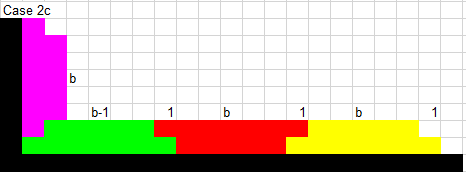Proving this scenario is also impossible takes a deeper, more complicated analysis. Here's my shot at it with a proof by contradiction. I hope it's possible to follow:

Let's assume it's possible to tile a rectangle with the shape (1, >=3, 1). Let's call the width of this rectangle W and the height H. The bottom-left corner will look like what you made, and the bottom-right corner will look similar. There is only one way to fill the bottom two row of the rectangle (see note 1): repeat the construction in the image above until the entire bottom two rows are filled.

After doing that, you have some sort of U-like shape. At the very left you have a tall polyomino with the base against the left wall. Let's call this polyomino a wall polyomino. At the very right of the rectangle is another wall polyomino. Now let's look at the third row from the bottom of the rectangle. The left two columns and the right two columns of this row are already occupied by the two wall polyominoes, but the rest still have to get tiled. Let's call this gap in the third row the canyon. Now look at the following situation: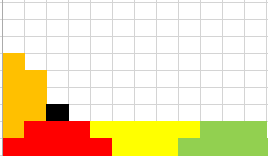The black square must get tiled. There are two ways to do it, and they are extremely similar to the two ways of tiling the bottom-left corner of the rectangle. The same is going in the bottom-right corner of the rectangle. Now the third row from the bottom of the rectangle. This one must get tiles entirely and the process of doing that is the same as the one for tiling the bottom row. There's only one difference: the width is no longer W. It's now W-4.

I'm pretty sure it's impossible to tile the entire third row, but let's give it the benefit of the doubt. Now it's required to tile the fifth row from the bottom. The leftmost four columns and the rightmost four columns are now occupied by wall polyominoes, so it's required to fill a canyon with a width of W-8.

Keep repeating the filling of the corners and the canyon and keep assuming it's possible to tile the canyon (even though I suspect it never is). Eventually you'll reach a canyon width of 4, 3, 2 or 1. Those canyons can never get tiled. They are too narrow to place a polyonimo horizontally and if you place a polyomino vertically, there will always be at least one tile in the canyon that doesn't get tiled.

So for any f(1, >=3, 1) it's impossible to tile a rectangle.

Note 1: technically there are multiple ways to tile the bottom row. It's also possible to place a few more wall polyominos somewhere in the middle of the width of the rectangle, but this merely creates different canyons. The proof of why these canyons can't get filled is very similar to the proof why the big canyon can't get filled.

- 1 year, 2 months ago

Ooh. True. I forgot that case

- 1 year, 2 months ago

Since the polyomino has an area of 10, the area of a rectangle that can be filled with the polynomial will have an area which is a multiple of 10. In order for this to be possible, the lengths of the rectangle must be multiples of certain numbers - if one of the side lengths was 10, then the area will always be a multiple of 10. If one of the side lengths was a multiple of 2 and the other was a multiple of 5, then the area will always be a multiple of 10. If the side lengths of a rectangle don't meet either of these conditions, then it cannot be filled with the polyomino.

(...did I seriously type "polynomial" instead of "polyomino"?)

- 1 year, 6 months ago

Agreed. But we need some casework to show that certain combinations of polyminos to fill the edges are not possible. Then, if the combinations necessary to reach an area divisible by 10 are proven impossible, the answer would be no.

- 1 year, 6 months ago

Also, using the checkboard argument, we can prove that an even number of the polyomino is needed, so the area must be a multiple of 20

- 1 year, 3 months ago

I wonder if it is possible to merge David's insight which limits the number of states each corners can be put in, with Scott's insight which seeks to formalizes the pattern that any valid string of blocks should follow, to figure out if there can exist any string of block at the edge such that the string starts with one of the possible corner block and also ends with one of the corner blocks.

- 1 year, 5 months ago

I took each connection in Scott Reynolds' comment and saw that the space between each polyomino in the second row in from the edge was either 0,1,2. Here's the list (it uses the same lettering as Scott's comment): 0: A->D ; B->C ; B->E ; C->B ; D->A ; E->F ; F->A 1: B->F ; E->A 2: A->E ; A->C ; B->A ; D->B ; F->B

This means you can't lay the polyominoes so that they inter-lock with the next row down, like here (the purple bit is an arbitrary tiling, and the bottom of it is an edge):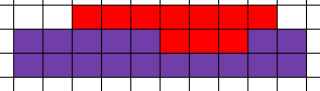This picture is impossible, regardless of how we fill in the purple part.

This means that the rectangle might look something like this close to one of the edges: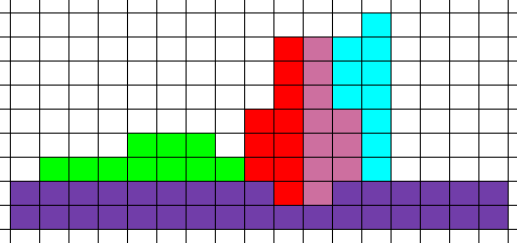- 1 year, 5 months ago

Picture Trick: If you go to a completely different problem that you solved correctly, click on the "discuss solutions" button, and write your comment in the "Add your Solutions" box, you can add pictures (and preview equations). But then instead of posting it there, copy your work and paste it in the discussion you want to comment in and post it there.

- 1 year, 5 months ago

Following on from comments from Daniel Stone and Loius Ullman, note that the number of tiles must be even and therefore the total area is divisible by 20 and the sides of the rectangle must have factors of two at least twice and five at least once. To see that the number of tiles is even, note that if the squares are coloured alternately back and white as on a chess board each tile covers two more white squares than black squares or vice versa. Since the total number of squares in the rectangle is even ( the area is a multiple of 10 (the area of the tile), there are the same number of black squares as white in the rectangle. Hence to cover the rectangle there must be the same number of tiles with more black squares as there are tiles with more white squares: that is the tiles come in pairs.

- 1 year, 5 months ago

Just a question: if you can construct a rectangle with some polyomino, is it necessarily true that the figure is rotationally symmetric?

- 1 year, 5 months ago

Because if so, we could determine all the corners of the assumed rectangle by setting only one of them from David Vreken's list of possible corners.

- 1 year, 5 months ago

I think the answer is yes, but I just want to be sure.

- 1 year, 5 months ago

@Daniel: Could you say what makes you think it must be rotationally symmetric? My intuition is that this is not true in general. If the polyomino is an ordinary domino [ = two squares ] then non-rotationally symmetric tilings certainly exist.

- 1 year, 5 months ago

Observation. Look at the example polyomino above: if you rotate it by 180°, you get the same arrangement. If you rotate by 90°, you get the same cornner arrangments. I haven't really tried to find one that is not like that. I'll get to it.

- 1 year, 5 months ago

In a previous post I showed that the number of pieces in a tiled rectangle was even. [In short the proof was that if the cells were coloured as on a chess board the number of pieces with more white squares must be the same as the number of pieces with more black squares as the rectangle contained the same number of black and white squares.] I now extend this result to show that the both the number of horizontal pieces and the number of vertical pieces in a tiled rectangle must be even. I also show that if one of the sides of the rectangle is odd, the the other side must be divisible by 8.

Let the rectangle be m squares horizonally by n squares vertically. Orientate the rectangle so that m is even.

Shade the rectangle with vertical stripes so that the odd numbered columns are white and the even numbered columns are grey. Since m is even there are the same number of white and grey squares.

Note that any tile placed horizontally will cover the same number of white and grey squares – 5 each. Hence all the horizontal tiles together will cover the same number of grey and white squares. The vertically placed tiles will cover the remaining squares which will also have the same number of white and grey squares.

Now a vertical tile will have 7 squares in one column and 3 squares in the adjacent column: it will therefore have either 4 more white squares than grey or 4 more grey squares than white. In order to cover the same number of grey and white squares there must be the same number of each of these two types of vertical tile. Hence the number of vertical tiles is even.

However, horizontal tiles is the total number of tiles (which is even) less the number of vertical tiles ( also even). Hence the number of horizontal tiles is even.

Now recolour the rectangle, with the rows alternately red and blue, with the odd numbered rows in red and the even numbered rows in blue.

There are two possibilities a) both m and n are even, or b) m is even and n is odd. Suppose the later: in this case we have one more red row than blue and there are m more red squares than blue. As before we note the vertical tiles contribute an equal number of red and blue squares. Let there be r horizontal tiles with more red than blue squares, and b horizontal tiles with more blue than red squares.

Let r = b + k

The number of horizontal tiles = r+b = 2b+k which we proved to be even. Hence k is even.

But the number of red squares more than blue covered by the horizontal tiles is (4r – 4b) and we know this to be m. Therefore 4r-4b = m, that is: 4 (b+k) – 4 b = 4k = m. But k is even, so m is divisible by 8.

Hence we have proved that if the rectangle can be tiled, either both sides of the rectangle are even or one side is divisible by 8.

- 1 year, 1 month ago

Since we already know that the area 4 S/Z polyomino can't be used to construct a rectangle, what if we assumed that a axb rectangle made of S/Z polyominoes existed, then proved by contradiction that it couldn't exist? If it works, we may be able to do the same to the area 10 polynomial we're using.

- 1 year, 5 months ago

Does anyone know of any examples of polynomioes that have been shown to not be able to tile a rectangle? I'd like to see what sort of proofs people use.

In particular, are there any polynomioes that have been found that are known to tile a half-strip but not a rectangle?

- 1 year, 2 months ago

https://brilliant.org/groups/open-problems-group/problems/pentomino-rectangle-tiling/

This question has an example of a polyomino that doesn't tile a rectangle.

There are also polyominoes that can't tile a rectangle, because there will always be a hole. For example, a 3x3 square that has a 1x1 hole in the center.

- 1 year, 2 months ago

Is the question to ask you to use a single polyomino to form a rectangle or a combination of them?

- 12 months ago

You are only allowed to use one type of polynomial. In this case using only the light blue one.

Staff - 11 months, 4 weeks ago

From the problem description: "The answer incidentally is thought to be "no", but nobody has proven it yet."

Why is the answer thought to be "no"? Is there previous research into this particular polyomino?

- 1 year, 2 months ago

There is no side of two blocks which is always required

- 1 year, 5 months ago

Could you please explain yourself a little better?

- 1 year, 5 months ago

What do you mean by this?

- 1 year, 5 months ago